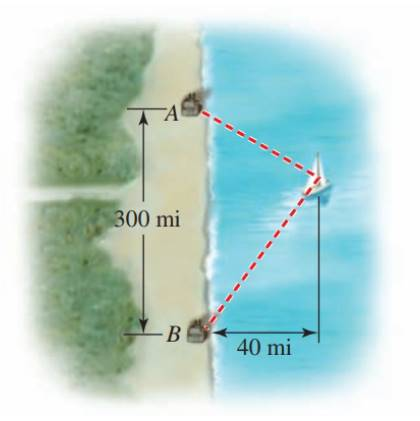Chapter 12.CR, Problem 66E### Algebra and Trigonometry (MindTap ...

4th Edition
James Stewart + 2 others
ISBN: 9781305071742

#### Solutions

Chapter
Section### Algebra and Trigonometry (MindTap ...

4th Edition
James Stewart + 2 others
ISBN: 9781305071742
Textbook Problem

# LORAN A ship is located 40 mi from a straight shoreline. LORAN stations are located at points A and B on the shoreline, 300 mi apart. From the LORAN signals, the captain determines that the ship is 80 mi closer to A than to B . Find the location of the ship. ( Place A and B on the y-axis with the x-axis halfway between them. Find the x − and y − coordinates of the ship.)To determine

To find:

The location of the ship.

Explanation

Given:

A ship is located 40 mi from a straight shoreline. LORAN stations are located at points A and B on the shoreline, 300 mi apart. From the LORAN signals, the captain determines that the ship is 80 mi closer to A than to B.

Approach:

Place A and B on the y-axis with the x-axis halfway between them as shown in the figure below.

Let x be the vertical distance between A and the ship and y be the vertical distance between B and the ship.

Figure (1)

By Pythagoras theorem,

x2+402=d2x=d21600

y2+402=(d+80)2y=(d+80)21600

x+y=300d21600+(d+80)21600=300 (1)

Simplify the equation.

For a quadratic equation of the form ax2+bx+c=0, the solutions are:

x=b±b24ac2a

Calculation:

From equation (1),

### Still sussing out bartleby?

Check out a sample textbook solution.

See a sample solution

#### The Solution to Your Study Problems

Bartleby provides explanations to thousands of textbook problems written by our experts, many with advanced degrees!

Get Started